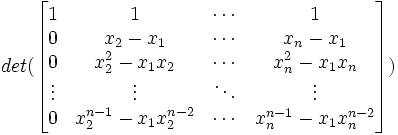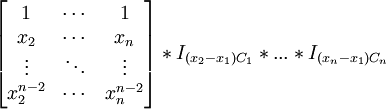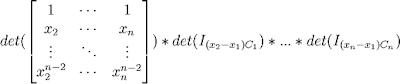## Tuesday, July 10, 2007

### Newton's Identities: Vandermonde Matrix

In today's blog, I will provide a formula for obtaining the determinant of the Vandermonde matrix. For review of determinants of n x n matrices, start here.

This blog is part of the discussion of Newton's Identities. If you are interested in going to the beginning of this thread, then start here.

Definition 1: Vandermonde matrix Vn

The Vandermonde matrix is an n x n matrix where the jth column is the vector (x1j-1, x2j-1, ..., xnj-1).

So based on this definition, the Vandermonde matrix Vn =Theorem 1:Proof:

(1) Using elementary matrices (see Lemma 3, here), we know that:

I(-x1R1 + R2)*I(-x2Rn-2 + R3)*...*I(-xn-1Rn-1 + Rn)*Vn =(2) Using a basic property of determinants of matrices (see Definition 4, here), we know that:

det(I(-x1R1 + R2)*I(-x2Rn-2 + R3)*...*I(-xn-1Rn-1 + Rn)*Vn) = det[I(-x1R1 + R2)]*det[I(-x2Rn-2 + R3)]*...*det[I(-xn-1Rn-1 + Rn)]*det(Vn)

(3) Now, since det(I(kR1 + R2)) = 1 (see Lemma 7, here), this gives us:

det(Vn) =(4) We can further simplify this expression to be:

det(Vn) = det(M1,1(Vn))

[See Corollary 3.1, here where M1,1 is the Minor of Ent1,1(Vn); see Definition 1 here for definition of the minor of a matrix; see Definition 1, here for definition of Ent1,1(Vn) notation]

(5) Now, we know that M1,1(Vn) ==(6) Using elementary matrices (see Corollary 4.2, here), we know that:

M1,1(Vn) =(7) Using step #4 gives us:

det(Vn) = det(M1,1(Vn) =(8) Since det(I(kCi)) = det(I(kRi) = k [see Theorem 9, here; see Lemma 4, here], we have:

det(Vn) =(9) So, we have found ourselves in the same situation as step#1 except that we have eliminated x1 as a column.

(10) If we repeat, the same process, we ultimately get:

det(Vn) =(11) So, using the determinant formula for 2 x 2 matrices (see Property 5, here; see Lemma 1, here), we have:QED

References